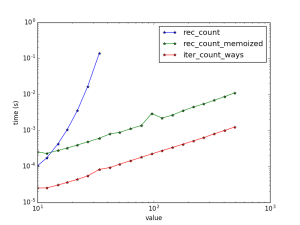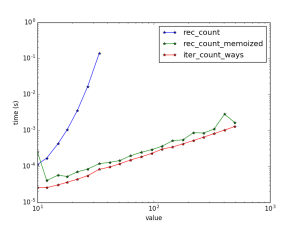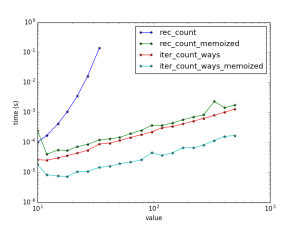Recursion is something that many computer science majors consider elegant. However, in simulation, speed far outweighs how many lines of code are underneath. [That is one reason why physicists still code in Fortran.]

I recently did some fooling around with recursive algorithms in Python. They are viewable at https://github.com/delton137/python_tests

To time different functions, I created a function for timing:

Next, a function for timing a list of functions of the form f(N) for different N and making a nice log-log plot:

Now let’s compare some ways of calculating the Fibonacci sequence. First we have the normal recursive method:

The problem with recursion is that it involves many redundant calls (see this this tree structure to see why), which causes the Fibonacci calculation to scale exponentially with N. This can be solved with memoization, which means you store previously calculated values in a lookup table (cache) so they can be used if the function is called again. In Python you can memoize a function with the memoization decorator:

Next we have simple iteration:

Next we have matrix representation:

Finally, just for fun, the direct computation:

now we run:

We obtainAs expected, the pure recursive method scales as exp(N). The memoized method linearly but uses significant memory [log(N)]. The iterative method has the same scaling but is almost 100x faster!

As a side note, the maximum recursion depth in Python is set very low. I had to use the following to increase it:

import sys
sys.setrecursionlimit(10000) 

Now let’s do another very common recursive problem – the ‘making change’ problem. Given a target amount N, how many ways are there to make change, if you have an unlimited number of coins with denominations in the set {1,5,10,25}? The recursive algorithm tries subtracting all possible combinations from the target amount and sees if any of them work:

The iterative solution starts from N=1, and then builds up to N=N:

Now let’s test them:

functs_to_test = [rec_count, rec_count_memoized, iter_count_ways]
plot_scaling(functs_to_test)


c<figcaption class="caption wp-caption-text">Speeds and scaling for coins problem.</figcaption></figure>

Now I should note that in these tests I am resetting the memoization cache for each new test N. If you don’t reset the cache and reuses it for each successive test, somewhat surprisingly one obtains only a slight speedup:I thought this may be due to the fact that the test points are logarithmically spaced, but it appears not.

Memoization makes recursion palatable, but it seems iteration is always faster.

It is worth mentioning that iterative methods can be memorized as well – any function that may be called repeatably can.  For instance, I memoized the iterative method: it keeps all the values it has computed so far, and then picks up where it left off when asked to compute a value that is not already stored:Although recursive methods run slower, they sometimes use less lines of code than iteration and for many are easier to understand. Recursive methods are useful for certain specific tasks, as well, such as traversing tree structures.